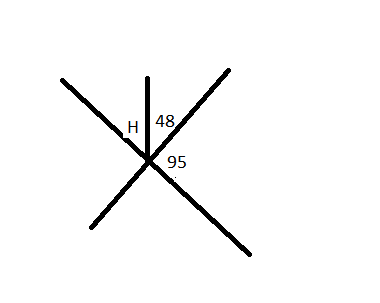chapter 1 Skills Maint 5
Define congruent *
Two angles are complementary. One angle is 4x - 4 and the other is 3x + 18. Solve for x. *
Two angles are vertical. One angles is 34 degrees and the other is 4x. Solve for x. *
Find the measure of angle H. The only answer accepted will be a number. so if you think H = 190, just put 190 as your answer *Period *